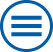## The group contributes to the following mathematical research topics of WIAS:

Analysis of Partial Differential Equations and Evolutionary Equations

Partial differential equations form an adequate and powerful instrument to provide a mathematical model for nature. At the Weierstrass Institute this research has two essential focuses: (a) Regularity for the solutions of linear elliptic equations and (b) Existence, uniqueness and regularity for evolution equations. [>> more]

Modeling, analysis and numerics of phase field models

A diffuse phase field model is a mathematical model for describing microstructural phenomena and for predicting morphological evolution on the mesoscale. It is applied to a wide variety of material processes such as solidification, coarsening in alloys, crack propagation and martensitic transformations. [>> more]

Multi scale modeling and hybrid models

Because of the ongoing miniaturization, modern devices in mechanics, electronics, or optics become smaller and smaller. Their working principles depend more and more on effects on different spatial scales. The aim is to optimize the efficiency by adapting the arrangement of interfaces or periodic microstructures. The understanding of the transfer between different scales relies on mathematical methods such as homogenization, asymptotic analysis, or Gamma convergence. The generated effective models are coupled partial differential equations combining volume and interfacial effects. [>> more]

Multiscale Modeling and Asymptotic Analysis

This work is focussed on plates, beams, shells and arches; sharp interface limits of generalized Navier-Stokes-Korteweg systems; sharp limits of regularized diffusion equations with mechanical coupling for applications in energy technology as well as thin film equations. [>> more]

Variational methods

Many physical phenomena can be described by suitable functionals, whose critical points play the role of equilibrium solutions. Of particular interest are local and global minimizers: a soap bubble minimizes the surface area subject to a given volume and an elastic body minimizes the stored elastic energy subject to given boundary conditions. [>> more]